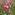Math GRE (39 Cards)
byNina
Front cards Back cards
1 Area of a Circle Pie R Squared
2 Area of a Rectangle Length times width
3 Area of a Square Side squared
4 Area of a Triangle Half the base, times the height
5 Average Sum of Terms, over, the Number of Terms
6 Circumference 2 pie R
7 Common denominator A denominator that is the same in two or more fractions
8 Composite number an integer that is greater than one that is not prime
9 Denominator The bottom number of a fraction.
10 Diameter 2 times the radius; 2R
11 Divisible A number is divisible by its factors; 60 is divisible by 1, 2, 3, 4, 5, 6, 10, 12, 15, 20, 30, and 60
12 Divisor Integers that may be multiplied to produce a number; numbers that an integer is divisible by; e.g. all divisors for 60 are 1, 2, 3, 4, 5, 6, 10, 12, 15, 20, 30, and 60
13 Equilateral triangle 60; 60; 60
14 Even integer An integer that is divisible by 2
15 Factor Integers that may be multiplied to produce a number; numbers that an integer is divisible by; e.g. all factors for 60 are 1, 2, 3, 4, 5, 6, 10, 12, 15, 20, 30, and 60
16 Fraction Is a number of the form a/b , where a and b are integers and b ≠ 0
17 Greatest common divisor The largest factor that two or more numbers have in common
18 integers the set of positive whole numbers and their opposites (negative numbers) and 0
19 Interger A positive or negative number
20 Invert The reciprocal of a fraction (turn 3/4 into 4/3)
21 least common multiple the smallest multiple that is exactly divisible by every member of a set of numbers
22 Median The middle term of a set in sequential order
23 mixed number a whole number , a whole number and a fractional part
24 Mode The term that occurs most frequently
25 Multiple The product created by a numbers factors; 25 is a multiple of -25, -5, -1, 1, 5, and 25
26 numerator the top number of a fraction
27 Odd integer An integer that is not divisible by 2
28 Prime divisors A list of prime numbers that produce by multiplication some number; the prime divisors for 12 are 2, 2, and 3 because 2 * 2 * 3 = 12
29 Prime factorization a list of the prime divisors
30 Prime number Natural number greater than 1 that has no positive divisor other than 1 and itself;
31 Probability The likelihood of an event occurring;
32 Pythagorean Triplets 3, 4, 5;
33 Quotient The integer obtained by division (always has a remainder even if that remainder is 0; 19 ÷ 7 = quotient 2 with a remainder of 5)
34 Range The difference between the greatest term and the least term
35 Rational numbers Any number that can be expressed as a fraction
36 Reciprocal The inverse of a fraction (3/4 becomes 4/3)
37 Remainder The remaining number after division (19 ÷ 7 = 2 with a remainder of 5)
38 Right Triangle - 30, 60, 90 X; X Square root of 3; 2X
39 Right Triangle - 45, 45, 90 X; X; X Square root of 2